# AP Chemistry : Atomic Mass

## Example Questions

### Example Question #1 : Atomic Mass

A certain element X is comprised of isotopes A, B, and C. Isotope A has a mass of 50 amu and is 70% of naturally occurring X. Isotope B is 35 amu and is 25% of X. Isotope C has a mass of 70 amu and is 5% of X. What is the atomic weight of element X?

55 g/mol

40 g/mol

47 g/mol

87 g/mol

70 g/mol

47 g/mol

Explanation:

0.70 (50 amu) + 0.25 (35 amu) + 0.05 (70 amu) = 47 amu = 47 g/mol

### Example Question #2 : Atomic Mass

The average molecular weight for element X is 59.97 g/mol. There are two known isotopes of element X, one weighing 59 g/mol, and the other weighing 61 g/mol. What is the relative abundance of each?

48.5% X-59, 51.5% X-61

50% X-59, 50% X-61

51.5% X-59, 48.5% X-61

75% X-59, 25% X-61

63% X-59, 37% X-61

51.5% X-59, 48.5% X-61

Explanation:

In this simplistic case, we only have two isotopes, so we know the following: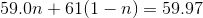Where "n" represents the relative abundance of X-59, and "(1-n)" is the relative abundance of X-61. We will algebraically solve for "n":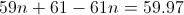simplifies to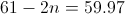simplifies to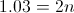which leads to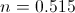If "n" is equal to 0.515, that means the relative abundance of X-59 is 51.5%, and thus the relative abundance of X-61 is 48.5%.

### Example Question #1 : Atomic Mass

Arbitrary element, X, has two isotopes,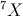and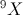. The mass listed on the periodic table is 8.000 grams per mole. Without doing any calculations, which isotope is more abundant?

There must be a third isotope in order for this to occur

They are both present in equal amountsis more prevalent

There is not enough information to answer this questionis more prevalent

They are both present in equal amounts

Explanation:

A calculation would show that the relative abundance of each is 50%, you can see that 8.000 g/mol is exactly the midpoint between the masses of 7 and 9, so each has to be present in equal quantities.

### Example Question #4 : Atomic Mass

An element has two main isotopes. The first isotope has a molar mass of 125 grams, and the second isotope has a molar mass of 128 grams. A naturally occurring sample of the element has a molar mass of 126.8 grams.

What is the lighter isotope's percentage in the natural sample?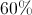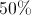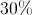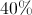Explanation:

In order to find the percentage of the lighter isotope in the natural sample, we need to use an equation that compares the percentage of each isotope and the natural molar mass. Since both of the isotopes together will account for 100% of the natural sample, we can say that the lighter isotope's percentage is, while the remaining percentage is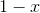.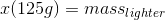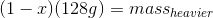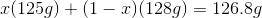Use this equation to solve for.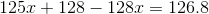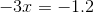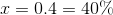In other words, we can conclude that the lighter isotope makes up 40% of the natural sample.

### Example Question #1 : Atomic Mass

Chemical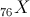has only two isotopes: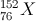and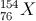. The atomic weight of chemical X, listed on the periodic table, is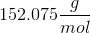. What is the relative abundance of each isotope?

The abundance ofis 96.25%, and the abundance ofis 3.75%

The abundance ofis 91.25%, and the abundance ofis 8.75%

The abundance ofis 50%, and the abundance ofis 50%

There is not enough information to answer this question

The abundance ofis 3.75%, and the abundance ofis 96.25%

The abundance ofis 96.25%, and the abundance ofis 3.75%

Explanation:

The abundance ofwill be referred to as "n," and the abundance ofis "1-n." Together, we know that these values account for the full abundance of chemical X in existence.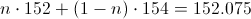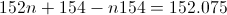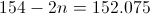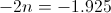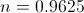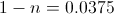The abundance ofis 96.25%, and the abundance ofis 3.75%.

### Example Question #1 : Atomic Mass

Many elements exist in nature as varying isotopes. Although one isotope commonly predominates due to stability, the other isotopes can affect the overall mass of one natural mole of the element.

Suppose a mystery element has two main isotopes: isotope X has a molar mass of 128 grams per mole and isotope Y has a molar mass of 132 grams per mole. One mole of this element, when found naturally, weighs 128.8 grams. What are the percentages of isotopes X and Y when found in nature?

50% X and 50% Y

80% X and 20% Y

20% X and 80% Y

60% X and 40% Y

80% X and 20% Y

Explanation:

This answer becomes a lot easier if we use some quick mental math. First, notice that if the two isotopes were equal in nature (50/50), than a mole would weigh 130 grams.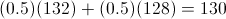Since the number is less than that, we can conclude that isotope X is more dominant than isotope Y, lowering the average mass. Now, we can use math to find the exact percentage.

Start by realizing that isotope X will make up some percentage of the element in nature (we will call this percentage "A") while isotope Y will make up the rest of that percentage (1-A).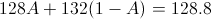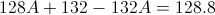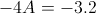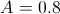This means that 80% of the element is composed of isotope X, and the other 20% is composed of isotope Y. Going back to our mental math, we see that, just as we predicted, isotope X is present in a larger amount than isotope Y.

### All AP Chemistry Resources